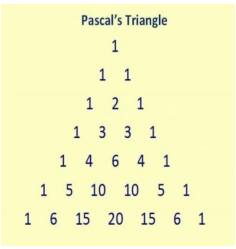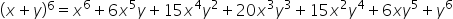Maths-
General
Easy

Question

# Use Pascal triangle to expand (x + y)6Hint:

## The correct answer is: 6Cr

### Step 1 of 1:The Pascal’s triangle is given by:From the triangle, the seventh line would give the coefficients of the expansion of the polynomial ( x + y)6  . Thus, we have:.

The expansion of the polynomial (x + y)6  can be found using the values of 6Cr#### With Turito Foundation.#### Get an Expert Advice From Turito.